## How to Calculate and Solve for Crack Opening Displacement | Polymer Deformation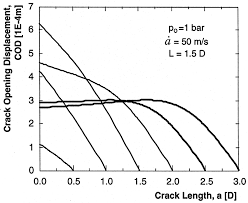The image above represents crack opening displacement.

To compute for crack opening displacement, two essential parameters are needed and these parameters are Shear Modulus (G) and Yield Strength (σys).

The formula for calculating crack opening displacement:

d = G / 2σys

Where:

d = Crack Opening Displacement (G)
G = Shear Modulus
σys = Yield Strength

Let’s solve an example;
Find the crack opening displacement when the shear modulus is 12 and the yield strength is 4.

This implies that;

G = Shear Modulus = 12
σys = Yield Strength = 4

d = G / 2σys
d = 12 / 2(4)
d = 12 / 8
d = 1.5

Therefore, the crack opening displacement is 1.5 m.

Calculating the Shear Modulus when the Crack Opening Displacement and the Yield Strength is Given.

G = d x σys

Where:

G = Shear Modulus
d = Crack Opening Displacement (G)
σys = Yield Strength

Let’s solve an example;
Find the shear modulus when the crack opening displacement is 10 and the yield strength is 4.

This implies that;

d = Crack Opening Displacement (G) = 10
σys = Yield Strength = 4

G = d x σys
G = 10 x 4
G = 40

Therefore, the shear modulus is 40.

## How to Calculate and Solve for Fracture Toughness on Knoop Indentor | Polymer Deformation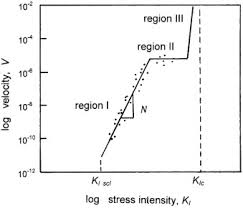The image above represents fracture toughness on knoop indentor.

To compute for fracture toughness on knoop indentor, five essential parameters are needed and these parameters are Indentor Constant (d), Elastic Modulus, (E), Indentation Hardness Value (H), Load (P) and a.

The formula for calculating fracture toughness on knoop indentor:

Kc = d(E)(P) / H(a)3/2

Where:

Kc = Fracture Toughness on Knoop Indentor
d = Indentor Constant
E = Elastic Modulus
H = Indentation Hardness Value
a

Let’s solve an example;
Find the fracture toughness on knoop indentor when the indentor constant is 2, the elastic modulus is 4, the indentation hardness value is 6, the load is 8 and a is 3.

This implies that;

d = Indentor Constant = 2
E = Elastic Modulus = 4
H = Indentation Hardness Value = 6
a = 3

Kc = d(E)(P) / H(a)3/2
Kc = (2)(4)(8) / (6)(3)3/2
Kc = 64 / (6)(5.196)
Kc = 64 / 31.176
Kc = 2.05

Therefore, the fracture toughness on knoop indentor is 2.05 J/m³.

## How to Calculate and Solve for Crack Opening Displacement | Polymer Deformation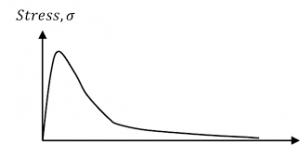The image above represents crack opening displacement.

To compute for crack opening displacement, three essential parameters are needed and these parameters are Fracture Toughness of Material (K), Elastic Modulus (E) and Yield Strength (σys).

The formula for calculating crack opening displacement:

d = / 2Eσys

Where:

d = Crack Opening Displacement (E)
K = Fracture Toughness of Material
E = Elastic Modulus
σys = Yield Strength

Let’s solve an example;
Find the crack opening displacement when the fracture toughness of material is 21, the elastic modulus is 11 and the yield strength is 7.

This implies that;

K = Fracture Toughness of Material = 21
E = Elastic Modulus = 11
σys = Yield Strength = 7

d = / 2Eσys
d = (21)² / 2(11)(7)
d = (441) / (154)
d = 2.86

Therefore, the crack opening displacement is 2.86 m.

## How to Calculate and Solve for Rod Toughness | Polymer Deformation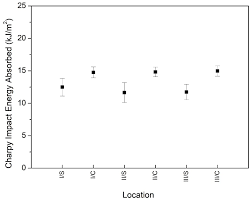The image above represents rod toughness.

To compute for rod toughness, three essential parameters are needed and these parameters are Short Rod Calibration Constant (A), Maximum Load (Pc) and Short Rod Diameter (B).

The formula for calculating rod toughness:

KIC = APcB-3/2

Where:

KIC = Rod Toughness
A = Short Rod Calibration Constant
B = Short Rod Diameter

Let’s solve an example;
Find the rod toughness when the short rod calibration constant is 12, the maximum load is 14 and the short rod diameter is 18.

This implies that;

A = Short Rod Calibration Constant = 12
Pc = Maximum Load = 14
B = Short Rod Diameter = 18

KIC = APcB-3/2
KIC = (12)(14)(18)-3/2
KIC = (12)(14)(0.013)
KIC = 2.199

Therefore, the rod toughness is 2.199 J/m³.

## How to Calculate and Solve for Apparent Length of Profile | Polymer DeformationThe image above represents apparent length of profile.

To compute for apparent length of profile, three essential parameters are needed and these parameters are Constant for Dimension of Length (Lo), Measurement Unit (η) and Fractal Dimension (D).

The formula for calculating apparent length of profile:

L(η) = Loη-(D – 1)

Where:

L(η) = Apparent Length of Profile
Lo = Constant for Dimension of Length
η = Measurement Unit
D = Fractal Dimension

Let’s solve an example;
Find the apparent length of profile when the constant for dimension of length is 10, the measurement unit is 2 and the fractal dimension is 4.

This implies that;

Lo = Constant for Dimension of Length =  10
η = Measurement Unit = 2
D = Fractal Dimension = 4

L(η) = Loη-(D – 1)
L(η) = 10(2)-(4 – 1)
L(η) = 10(2)-(3)
L(η) = 10(2)-3
L(η) = 10(0.125)
L(η) = 1.25

Therefore, the apparent length of profile is 1.25 m.

## How to Calculate and Solve for Linear Roughness Parameter I Polymer Deformation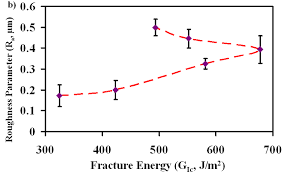The image above represents linear roughness parameter.

To compute for linear roughness parameter, two essential parameters are needed and these parameters are True Crack Length (Lt) and Projected Crack Length (Lp).

The formula for calculating linear roughness parameter:

RL = Lt / Lp

Where:

RL = Linear Roughness Parameter
Lt = True Crack Length
Lp = Projected Crack Length

Let’s solve an example;
Find the linear roughness parameter when the true crack length is 5 and the projected crack length is 8.

This implies that;

Lt = True Crack Length = 5
Lp = Projected Crack Length = 8

RL = Lt / Lp
RL = 5 / 8
RL = 0.625

Therefore, the linear roughness parameter is 0.625.

Calculating the True Crack Length when the Linear Roughness Parameter and the Projected Crack Length is Given.

Lt = RL x Lp

Where:

Lt = True Crack Length
RL = Linear Roughness Parameter
Lp = Projected Crack Length

Let’s solve an example;
Find the true crack length when the linear roughness parameter is 8 and the projected crack length is 2.

This implies that;

RL = Linear Roughness Parameter = 8
Lp = Projected Crack Length = 2

Lt = RL x Lp
Lt = 8 x 2
Lt = 16

Therefore, the true crack length is 16.

## How to Calculate and Solve for Surface Roughness Parameter I Polymer Deformation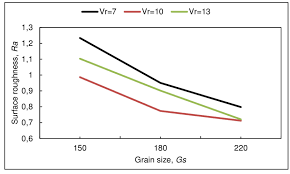The image above represents surface roughness parameter.

To compute for surface roughness parameter, one essential parameter is needed and this parameter is Linear Roughness Parameter (RL).

The formula for calculating surface roughness parameter:

RS = 4/π(RL – 1) + 1

Where:

RS = Surface Roughness Parameter
RL = Linear Roughness Parameter

Let’s solve an example;
Find the surface roughness parameter when the linear roughness parameter is 12.

This implies that;

RL = Linear Roughness Parameter = 12

RS = 4/π(RL – 1) + 1
RS = 4/π(12 – 1) + 1
RS = (1.273)(11) + 1
RS = 14.00 + 1
RS = 15.00

Therefore, the surface roughness parameter is 15.00.

## How to Calculate and Solve for Stress Damage Resistance | Polymer Deformation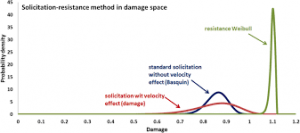The image above represents stress damage resistance.

To compute for stress damage resistance, four essential parameters are needed and these parameters are Elastic Modulus (E), Surface Fracture Energy (γ), Fracture Stress (σf) and Poisson’s Ratio (μ).

The formula for calculating stress damage resistance:

R”” = /σf² (1 – μ)

Where:

R”” = Stress Damage Resistance
E = Elastic Modulus
γ = Surface Fracture Energy
σf = Fracture Stress
μ = Poisson’s Ratio

Let’s solve an example;
Find the stress damage resistance when the elastic modulus is 21, the surface fracture energy is 7, the fracture stress is 12 and the poisson’s ratio is 4.

This implies that;

E = Elastic Modulus = 21
γ = Surface Fracture Energy = 7
σf = Fracture Stress = 12
μ = Poisson’s Ratio = 4

R”” = /σf² (1 – μ)
R”” = (21)(7)/(12)² (1 – 4)
R”” = (147)/(144) (-3)
R”” = (1.0208) (-3)
R”” = -3.06

Therefore, the stress damage resistance is -3.06.

## How to Calculate and Solve for Thermal Stress with Quenching | Polymer Deformation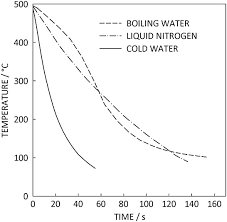The image above represents thermal stress with quenching.

To compute for thermal stress with quenching, six essential parameters are needed and these parameters are Fraction of Specific Geometry (C), Biot Quenching Modulus (β), Elastic Modulus (E), Linear Coefficient of Thermal Expansion (α), Change in Temperature (ΔT) and Poisson’s Ratio (μ).

The formula for calculating thermal stress with quenching:

σ = CβEαΔT / 1 – μ

Where:

σ = Thermal Stress with Quenching
C = Fraction of Specific Geometry
b = Biot Quenching Modulus
E = Elastic Modulus
α = Linear Coefficient of Thermal Expansion
ΔT = Change in Temperature
μ = Poisson’s Ratio

Let’s solve an example;
Find the thermal stress with quenching when the fraction of specific geometry is 1, the biot quenching modulus is 2, the elastic modulus is 3, the linear coefficient of thermal expansion is 4, the change in temperature is 5 and the poisson’s ratio is 6.

This implies that;

C = Fraction of Specific Geometry = 1
b = Biot Quenching Modulus = 2
E = Elastic Modulus = 3
α = Linear Coefficient of Thermal Expansion = 4
ΔT = Change in Temperature = 5
μ = Poisson’s Ratio = 6

σ = CβEαΔT / 1 – μ
σ = (1)(2)(3)(4)(5) / 1 – 6
σ = 120 / -5
σ = -24

Therefore, the thermal stress with quenching is -24 Pa.

## How to Calculate and Solve for Thermal Stress in Relation to Poisson’s Ratio | Polymer Deformation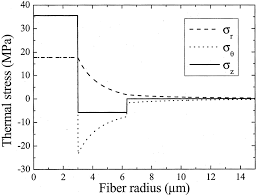The image above represents thermal stress in relation to poisson’s ratio.

To compute for thermal stress in relation to poisson’s ratio, four essential parameter are needed and these parameters are Elastic Modulus (E), Linear Coefficient of Thermal Expansion (α), Change in Temperature (ΔT) and Poisson’s Ratio (μ).

The formula for calculating thermal stress in relation to poisson’s ratio:

σ = EαΔT / 1 – μ

Where:

σ = Thermal Stress in Relation to Poisson’s Ratio
E = Elastic Modulus
α = Linear Coefficient of Thermal Expansion
ΔT = Change in Temperature
μ = Poisson’s Ratio

Let’s solve an example;
Find the thermal stress in relation to poisson’s ratio when the elastic modulus is 2, the linear coefficient of thermal expansion is 7, the change in temperature is 9 and the poisson’s ratio is 11.

This implies that;

E = Elastic Modulus = 2
α = Linear Coefficient of Thermal Expansion = 7
ΔT = Change in Temperature = 9
μ = Poisson’s Ratio = 11

σ = EαΔT / 1 – μ
σ = (2)(7)(9) / 1 – 11
σ = 126 / -10
σ = -12.6

Therefore, the thermal stress in relation to poisson’s ratio is -12.6 Pa.

Calculating the Elastic Modulus when the Thermal Stress in Relation to Poisson’s Ratio, the Linear Coefficient of Thermal Expansion, the Change in Temperature and the Poisson’s Ratio is Given.

E = σ(1 – μ) / αΔT

Where:

E = Elastic Modulus
σ = Thermal Stress in Relation to Poisson’s Ratio
α = Linear Coefficient of Thermal Expansion
ΔT = Change in Temperature
μ = Poisson’s Ratio

Let’s solve an example;
Find the elastic modulus when the thermal stress in relation to poisson’s ratio is 10, linear coefficient of thermal expansion is 2, the change in temperature is 3 and the poisson’s ratio is 4.

This implies that;

σ = Thermal Stress in Relation to Poisson’s Ratio = 10
α = Linear Coefficient of Thermal Expansion = 2
ΔT = Change in Temperature = 3
μ = Poisson’s Ratio = 3

E = σ(1 – μ) / αΔT
E = 10(1 – 3) / (2)(3)
E = 10(-2) / 6
E = -20 / 6
E = -3.33

Therefore, the elastic modulus is -3.33.